# CREST Reasoning Olympiad Class 8 Sample Papers

REGISTER NOW

## Syllabus:

Section 1: Series Completion and Inserting the Missing Character, Analogy and Classification, Blood Relations, Coding-Decoding, Direction Sense Test, Logical Venn Diagram, Alpha Numeric Sequence Puzzle, Number, Ranking and Time Sequence Test, Logical Sequence of Words, Alphabet Test, Mathematical Operations, Analytical Reasoning, Mirror Images and Water Images, Embedded Figures, Figure Formation, Construction of Squares, Grouping of Identical Figures, Figure Matrix, Paper Folding and Paper Cutting, Cubes and Dice, Dot Situations.

Achievers Section: Higher Order Thinking Questions - Syllabus as per Section 1

 Q.1 In the following question, select the related figures from the given alternatives.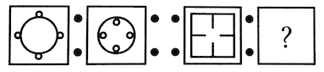Q.2 How many squares are there in the following figure?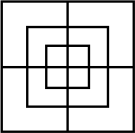Q.3 How many rectangles are there in the figure given below?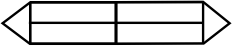Q.4 Which of the following will be the correct mirror image of the given figure, when the mirror is placed vertically to the right?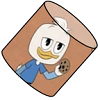Q.5 If RAID is written as %#©\$ and RIPE is coded as %©@*, then DEAR is coded as ____________.
 Q.6 Choose the missing number to complete the given series.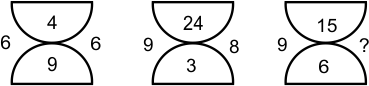Q.7 In a certain code, 'R' is '%', 'E' is '#', 'D' is '@' and 'A' is 'Δ'. How is 'DARE' written in that code?
 Q.8 Rajesh is facing west. He turns 45° in the clockwise direction and then another 180° in the same direction and then 270° in the anticlockwise direction. Which direction is he facing now?
 Q.9 In the following question, choose the correct mirror image of the given figure (X), if a mirror is placed vertically on the left side of the figure.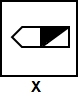Q.10 From the given suitable options, find the water image of the object given in the question figure.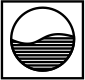Sample PDF of CREST Reasoning Olympiad for Class 8: